Homework Assignment 1 Due September 26, 2001

William Stein

Date: Math 124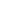HARVARD UNIVERSITYFall 2001

Instructions: Please work in groups, and acknowledge those you work with in your write up. Some of the problem below, such as factor a number'' can be quickly done with a computer. Feel free to do so, unless otherwise stated.

1. Let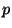be a prime number and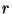and integer such that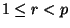. Prove thatdivides the binomial coefficient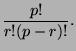You may not assume that this coefficient is a integer.

2. Compute the following gcd's using a pencil and the Euclidean algorithm: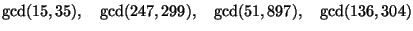3. Using mathematical induction to prove that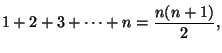then find a formula for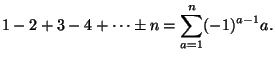4. What was the most recent prime year? I.e., which of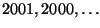was it?

5. Use the Euclidean algorithm to find integers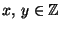such that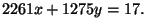[I did not tell you how to do this; see §1.8 of Davenport's book.]

6. Factor the year that you should graduate from Harvard as a product of primes. E.g., frosh answer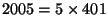.

7. Write a PARI program to print Hello Kitty'' five times.8. Let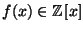be a polynomial with integer coefficients. Formulate a conjecture about when the set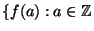and $f(a)$ is prime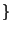is infinite. Give computational evidence for your conjecture.

9. Is it easy or hard for PARI to compute the gcd of two random 2000-digit numbers?

10. Prove that there are infinitely many primes of the form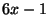.

1. Use PARI to compute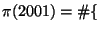primes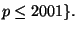2. The prime number theorem predicts that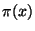is asymptotic to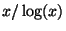. How close is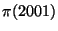to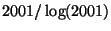?